# Motion 2

At 10 o'clock started from place A car speed at 90 km/h. 20 minutes after started from the place B at speed 60 km/h another car. Distance places A and B is 120 kilometers. What time and where they meet?

Result

t = 10:56 hodx =  84 km

#### Solution:

90(t-10)+60(t-(10+20/60))=120
x =90(t-10)

90•(t-10)+60•(t-(10+20/60))=120
x =90•(t-10)

9000t = 98400
90t-x = 900

t = 16415 ≈ 10.933333
x = 84

Our linear equations calculator calculates it.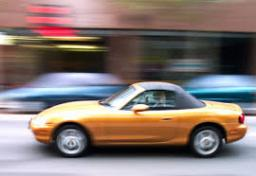We would be pleased if you find an error in the word problem, spelling mistakes, or inaccuracies and send it to us. Thank you!Tips to related online calculators
Do you want to convert length units?
Do you want to convert velocity (speed) units?
Do you want to convert time units like minutes to seconds?

## Next similar math problems:

• Tourists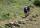From the cottage started first group of tourists at 10:00 AM at speed 4 km/h. The second started after them 47 minutes later at speed 6 km/h. How long and how many kilometers from the cottage will catch the first group?
• Two carsTwo cars started against each other at the same time to journey long 346 km. The first car went 53 km/h and the second 53 km/h. What distance will be between these cars 20 minutes before meet?
• Train from BrnoFirst train started from Brno at 8 am at 60 km per hour. Second train started from Opava at 7 o'clock at the speed of 40 kilometers per hour. In how many hours and how far from cities trains meet when the distance of cities is 200 kilometers.
• Znojmo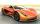From Znojmo to Brno started truck with a trailer at an average speed 53 km per hour. Against him 14 minutes later from Brno started car with an average speed of 1.2-times greater than the truck. How long and how far from Znojmo, they meet if the distance
• Cars 2Opava is 360 km distance from Prešov. At 8:00 hour two cars started against each other from this cities at average speeds 88 km/h and 121 km/h. At what hour its will meet and what distance its travel if car started from Prešov is faster?
• Cars motion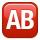From point A car start at the speed of 90 km/h. Against it departs from the point B at the same time another car at 60 km/h. For how long and where they met if the distance between A and B is 180 km?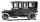Car started at 11:40 at speed 54 km/h from A and to B arrived at 12:20. Determine the distance A and B.
• Cars speedsDistance from city A to city B is 108 km. Two cars were simultaneously started from both places. The speed of a car coming from city A was 2 km/h higher than the second car. What was the speed of each car if they met in 54 minutes?
• Two shipsThe distance from A to B is 300km. At 7 am started from A to B a ferry with speed higher by 20 km/h than a ship that leaves at 8 o'clock from B to A. Both met at 10h 24min. Determine how far they will meet from A and when they reach the destination.
• Train from PragueFirst train from Prague started at 8:00 hour at 40 kilometers per hour. Train from Ostrava started at 9:20 at 80 km per hour. In how many hours and how far from cities with trains meet if the distance of cities is 400 km.
• TruckIn 7 hours started from town Krnov truck at speed 40 km/h. Passenger car started against it in 8 hours 30 minutes from the city of Jihlava at speed 70 km/h. Distance between this two cities is 225 km. At what time and at what distance from Krnov this two
• Reconstruction of the corridorCalculate how many minutes will be reduced to travel 187 km long railway corridor, where the maximum speed increases from 120 km/h to 160 km/h. Calculate how many minutes will shorten travel time, if we consider that the train must stop at 6 stations, eac
• Two trains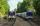Two trains go at each other. First at speed 40 km/h and the second at speed 60 km/h. At what distance will be 30 minutes before they will meet?
• Pedestrian and the cyclist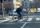Pedestrian and cyclist went against each from the two cities that are distant 40 km. Pedestrian started 30 minutes before the cyclist and walk an average speed of 5 km/h. What speed went cyclist when met after 120 minutes from the start of the pedestrian?
• Motorcyclist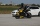From Trutnov, the motorcyclist started at an average speed of 60km/hour. At 12.30hrs the passenger car was started at a speed of 80km/hour. How many hours and at what distance from Trutnov will car catch a motorcycle?
• Cars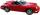Cars started at 7:00 AM. From point A car run at 40 km per hour, from B car run 70 km per hour. Between A and B is 250 km long road. When and where will meet (measured from point A)?
• Two citiesThe distance between cities A and B is 132 km. At 9.00 am, the cyclist started the bike at an average speed of 24 km/h, and at 10.00 h started from the B cyclist at an average speed of 30 km/h. How long and far from A will they both meet?